# 2018 ACM-ICPC 南京赛区小结 By SmartLy @ Reconquista

## Statistics

• TYPE: Onsite Contest
• NAME: 2018 - ICPC Regional - Asia EC - Nanjing
• PLAT: PC^2
• TIME: 2018/10/15 09:00-14:10 CST
• LOCA: Nanjing University of Aeronautics and Astronautics
• TEAM: Reconquista [lsmll, jiangshibiao, lzw4896s]
• RANK:
• 10/326 3.06% (Including unofficial teams)
• University rank: 3 (Official teams only)
• WF advancement rank: 2
• SOLVE: 8/13
• PENALTY: 905
◦ A - 25
◦ B - 102
◦ E - (-5)
◦ G - 78 (+1)
◦ I - 45
◦ J - 29
◦ K - 194
◦ L - 235
◦ M - 157 (+1)

## Day -1

周五训练有点遗憾，又是赛后 $$1min$$ 过题。打完后颜学长在 $$Mojito$$ 请客。我吃了两个牛肉卷竟然就饱了。
晚上我把大家各自的模板去打印店打印了一下。睡得很晚，在猝死边缘试探。

## Day 0

五点半大力起床，甚至还去洗了衣服。整个书包都献给了模板，好重呀。
紫金港有 $$1+3+3=7$$ 个人打车。约好 $$6:15$$ 食堂集合，我预订了 $$6:20$$ 的车——结果 $$6:16$$ 竟然还是第二个到的？哈哈哈 $$Sunstar$$ 有个队员睡过头了，心疼等了十几分钟的出租车司机。啊啊啊我又把发票又丢了，哭哭。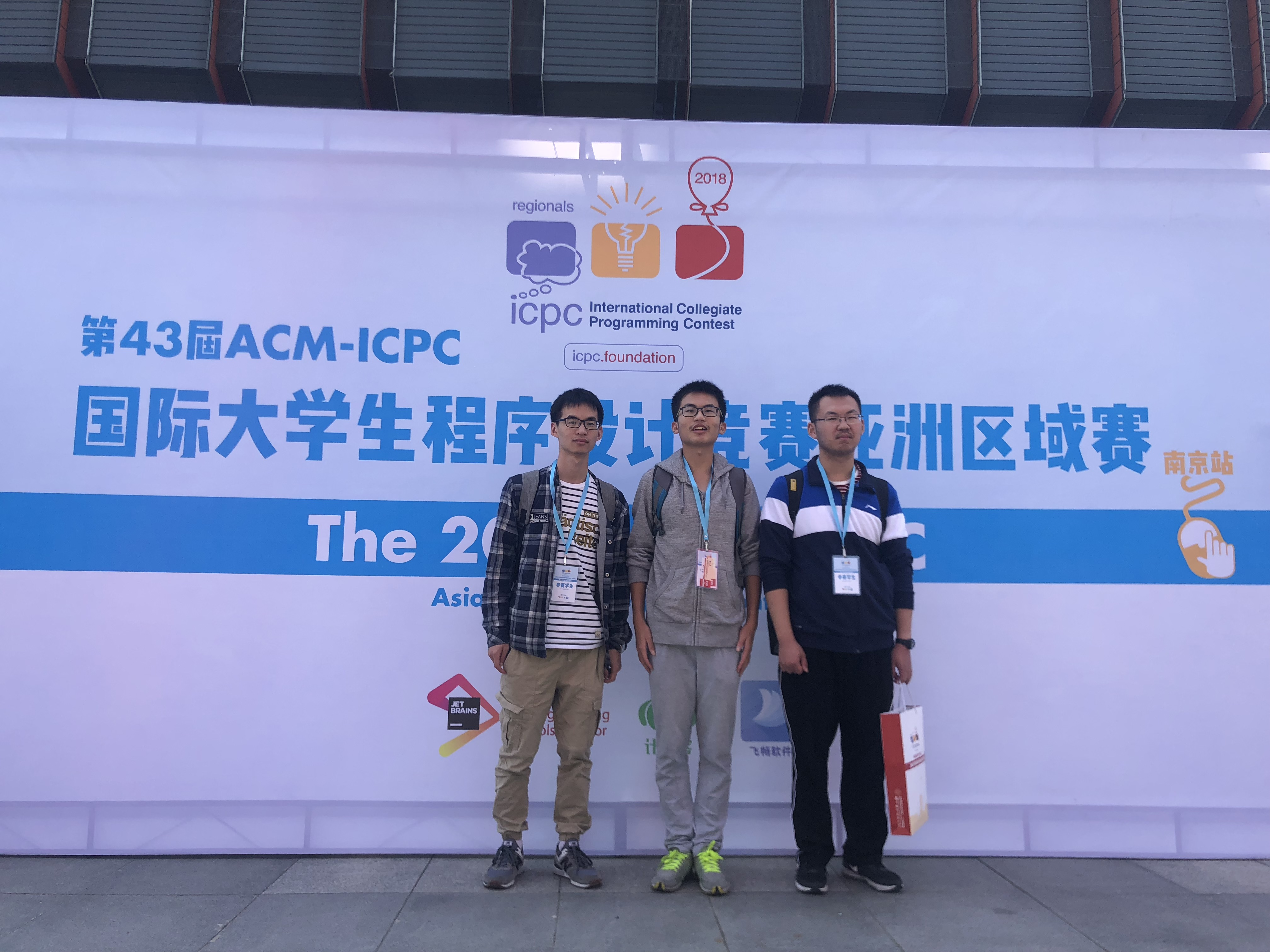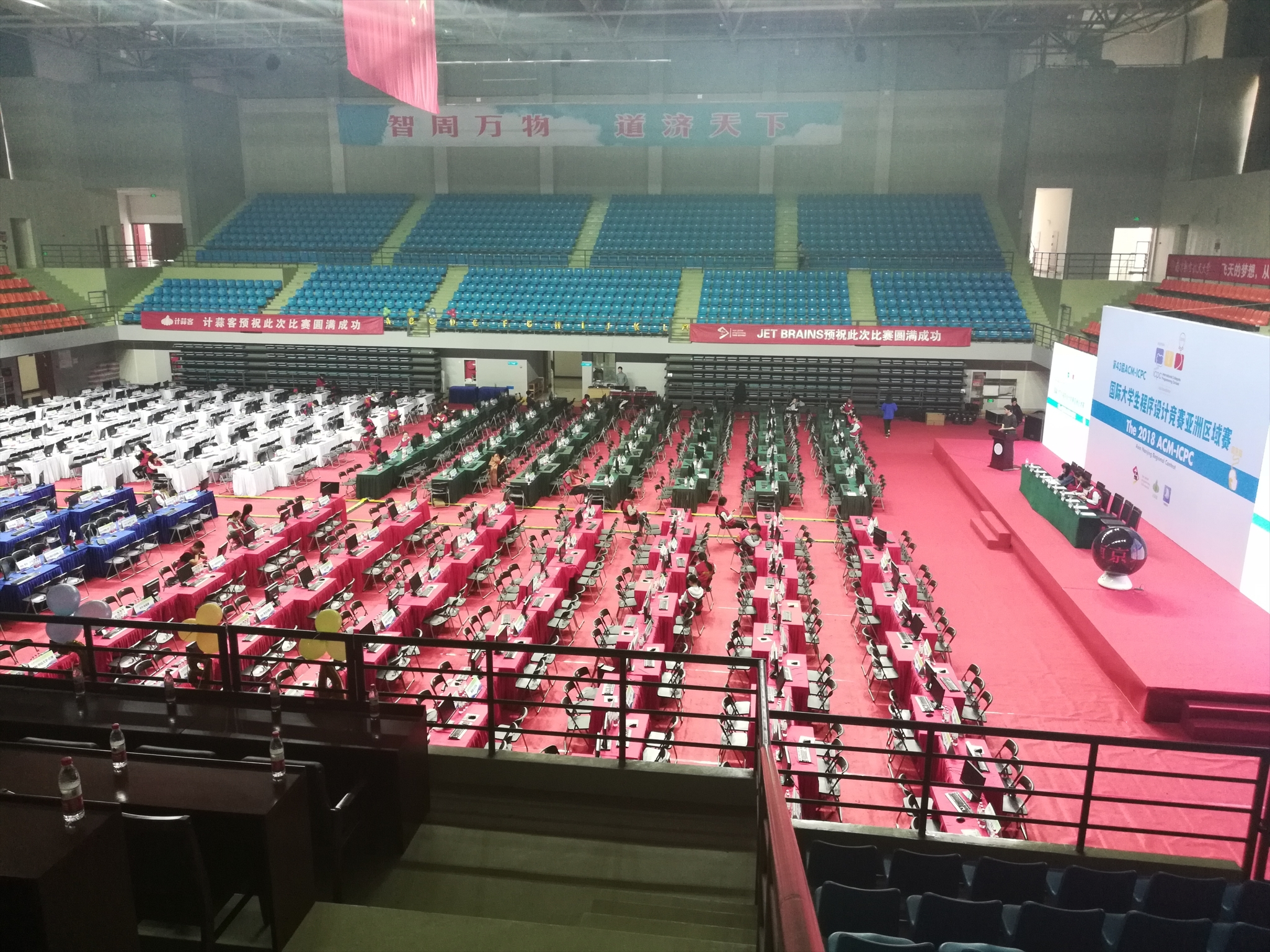到学校早了点，拍完照签完到就在体育馆椅子上傻坐着。 　　南航的食堂是真的厉害。午餐的种类、规模、口味，甚至是装修，都是一流的。有一种刘姥姥进大观园的感觉。 　　 　　热身赛有点糟糕啊。机子里没有 $gedit$，我们也就无法添加一键编译的命令了。即使用默认的 $mousepad$ 写程序，一按 $ctrl+S$ 就会卡好几秒。开始的半个小时里，$pc^2$ 还无法登陆。

四道陈题，并不是很难。$$A$$ 题听说很多队读错了题意，$$lsmll$$ 学长一发看懂一发通过有点帅啊。$$D$$ 题机械地把线段树和类欧给拼在一起了，让人担心第二天的比赛是不是也会是算法的堆砌。
在热身赛的赛场上，忽然切切实实感受到了紧张。时间过得真快呢，突然就在区域赛的现场了！
拿了 $$rk1$$ 反而有点担心呢。后来随便测了测 $$python$$$$int128$$，感觉还行。$$lzw$$ 接受了采访，聊到了发际线hhhhh。

傍晚叫上了中大的 $$rfy$$，北大的 $$syc$$，南大的 $$zyb$$，一起去新街口德基广场的 南京大牌档 吃饭。人好多呀，提前预约还等了很久。
店内的装饰古色古香，菜也的确挺好吃。菜量很小，看上去点了好几个，很快就吃完了。 $$22$$ 元一个的蟹黄包让汁水都流出来了，炒鸡心疼。点了两碗和鸭血有关的菜，感觉和老家的也没啥区别嘛。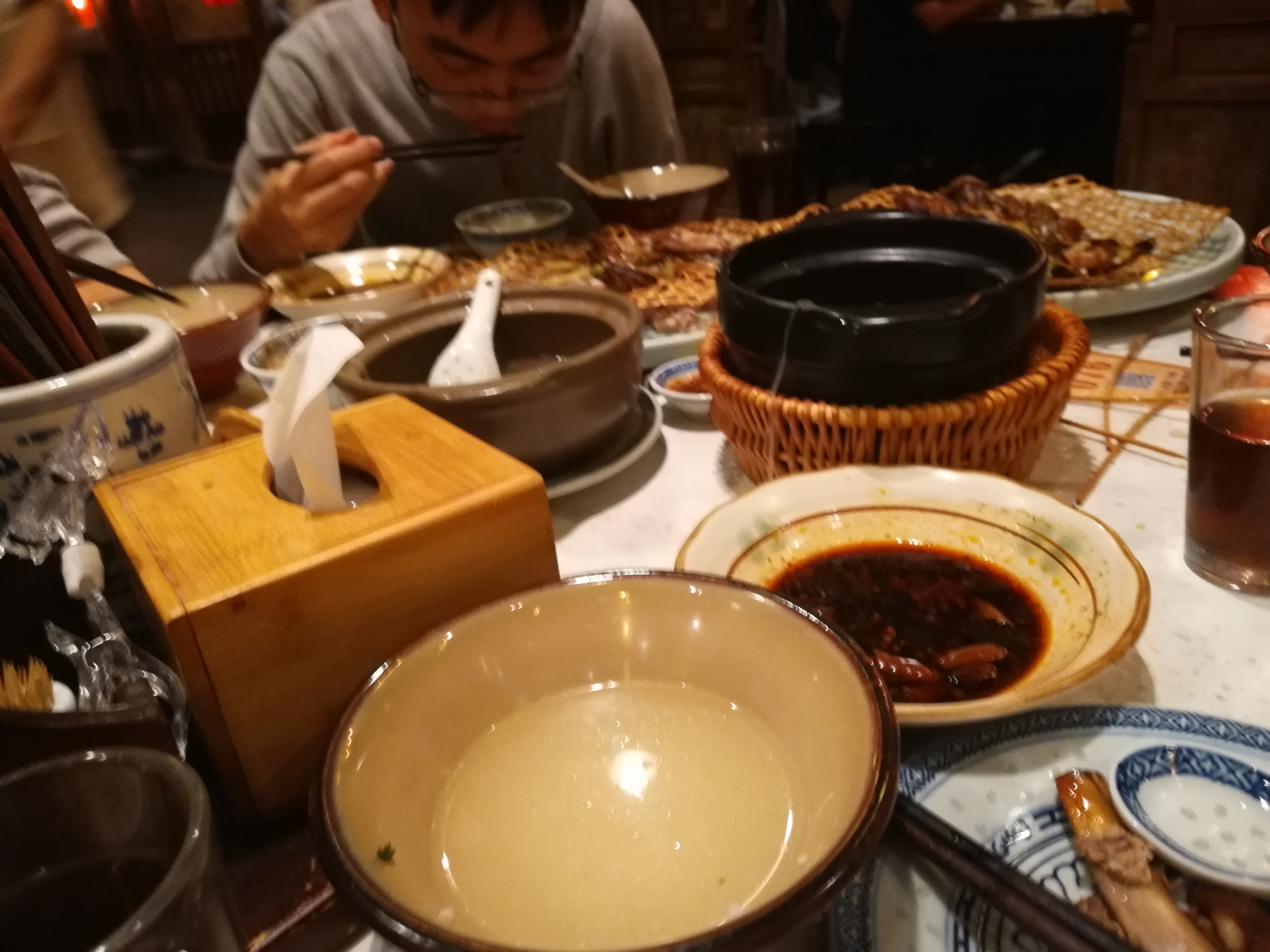整一天都被手机没电支配着。陆续借了三个没什么电的充电宝续命，最后安全赶到汉庭酒店。和帅气的 $$hyy$$ 学长一间(#.#)。
晚上在研究如何导入QQ漫游聊天记录，甚至开了SVIP，依旧没有捣鼓出来o(╥﹏╥)o。
前排仰慕 $$cjb$$，潇洒往返南京杭州！
晚上在 $$cjb$$ 房里各种分析局势，感觉很迷。到了十二点才肯睡觉，而且一直处于心慌慌的状态。

## Day 1

七点钟很不情愿地起床，感觉好困好困……早饭在KFC吃好像是标配？
站在体育馆前ICPC的宣传牌前发呆。胖颖的一句“陪着你”感觉好幸福鸭。
主办方挺有诚意，努力地解决热身赛里的问题。临时加好了 $$gedit$$，也流畅了很多，有点爽。

以下流水账基于 $$lsmll$$ 学长的游记。
开场从中间看，题都挺短，但没有裸签到，只找到了一道网络流的题。突然博弈题 $$A$$ 陆续有人过，可是看上去不是很水？思考了一会决定还是打表找规律。写得有点坎坷，感觉规律不明显啊。这时 $$A$$ 被屠榜，$$J$$ 也好多了。感觉内心有点慌，努力不表现出来。看了看 $$J$$ 是水题，我先去写了。$$A$$ 原来是表错了，得到大概结论后我们细致地讨论了细节，$$A1y25$$。紧接着我的 $$J1y29$$。丢给颜学长写 $$I$$ 题的网络流，$$I1y45$$。期间我和 $$lzw$$ 在做 $$G$$ 的计数，我们一人一种情况，假装很完美地找到了计算式。最后程序拼了拼，喜获 $$WA$$。这是又有点陷入僵局，后来经 $$lzw$$ 提醒，原来这题可以打表！打完一查数列表，稳了，$$G 2y78$$$$D$$ 被屠版了，我们一合计，发现二分后是判球是否有交点，感觉没这个板子。我看了看别的题也没思路，就果断上机写了个模拟退火。调了调参数（确保不TLE）自信一交，$$D1y102$$。写的时候，队友讨论出了 $$M$$，我稍加确认就继续写了。这道字符串要用 $$manacher$$$$ex-kmp$$，前者我是手写的，后者交给 $$lzw$$ 抄模板。写完轻松过样例啊，感觉稳如泰山，竟然TLE了？此时 $$lzw$$$$lsmll$$ 猜测了一种 $$K$$ 的做法，我就让出了机位，对着打印纸看得心浮气躁，也没看出啥错误。因为马拉车没抄板子，$$lzw$$ 提议把这部分也换成板子再交交看。我正动手着，$$lzw$$ 发现自己抄错了一个地方（而刚才我和他都没看出来呜呜呜），改了改 $$M2y157$$。颜学长超稳啊，说 $$K$$ 细节有点多，调完直接过了，$$K1y194$$。这段时间里，威威大力 $$carry$$，想到了 $$L$$ 题的一步关键转化。随后我们不断 $$fix$$ 这个模型，大致想到了做法，但还有一个小细节处理不好。$$lsmll$$ 过了后，提出了一种很简单的特判方法，我就去写了。思路挺清晰，最后 $$L1y235$$。此时我们还在轮流思考剩下有人过的这三题。其中 $$H$$$$E$$ 是智商/需要讨论的题，而过的人更多一点的 $$B$$ 像是个套路题。我纠结了一会 $$B$$ ，最终还是放弃了 。$$lzw$$ 去打了 $$E$$ 的表，但好像没什么规律。我突然想到了一步关键转化，然后大致会做了。当时还剩 $$20min$$，我想再确认一下细节，就让颜学长先去写起来。我把写法搞得更精简后，感觉颜学长没有完全理解，写得有点复杂，一时间很慌。此时主办方突然宣布：因技术问题加时$$10min$$。于是我提出还是我来重新写一写。最后三分钟编译通过，最后一分钟交了过样例后的版本。可惜最终没过，有点伤心。

最紧张的还是结束后的那段时间吧。反复核对封榜前的榜单，一遍遍地检查会造成威胁的队伍。比赛中发的零食根本没有动过。咖啡鸡还是niub啊，暴踩我们两题！最终是 WF advancement $$rk 2$$，还算宽心。被落日余晖同罚时碾压有点不爽，下回北京复仇啊啊啊。
$$Sunstar$$ 太可惜了，银牌第一o(╥﹏╥)o，没吃到 $$zhh$$ 学长的 $$bg$$ 。心疼 $$Yasha$$……

比赛里打了鸡血，整个下午都处于一种亢奋的状态。
因蜜汁原因滚榜取消，颁奖也就失去了乐趣= =。怎么只有冠军才有奖杯，气鼓鼓= =。

南大的猥琐小哥哥们收集起所有饭票（约摸有个两三百吧）径直去小卖部搬饮料了。有点niub啊，学不来学不来。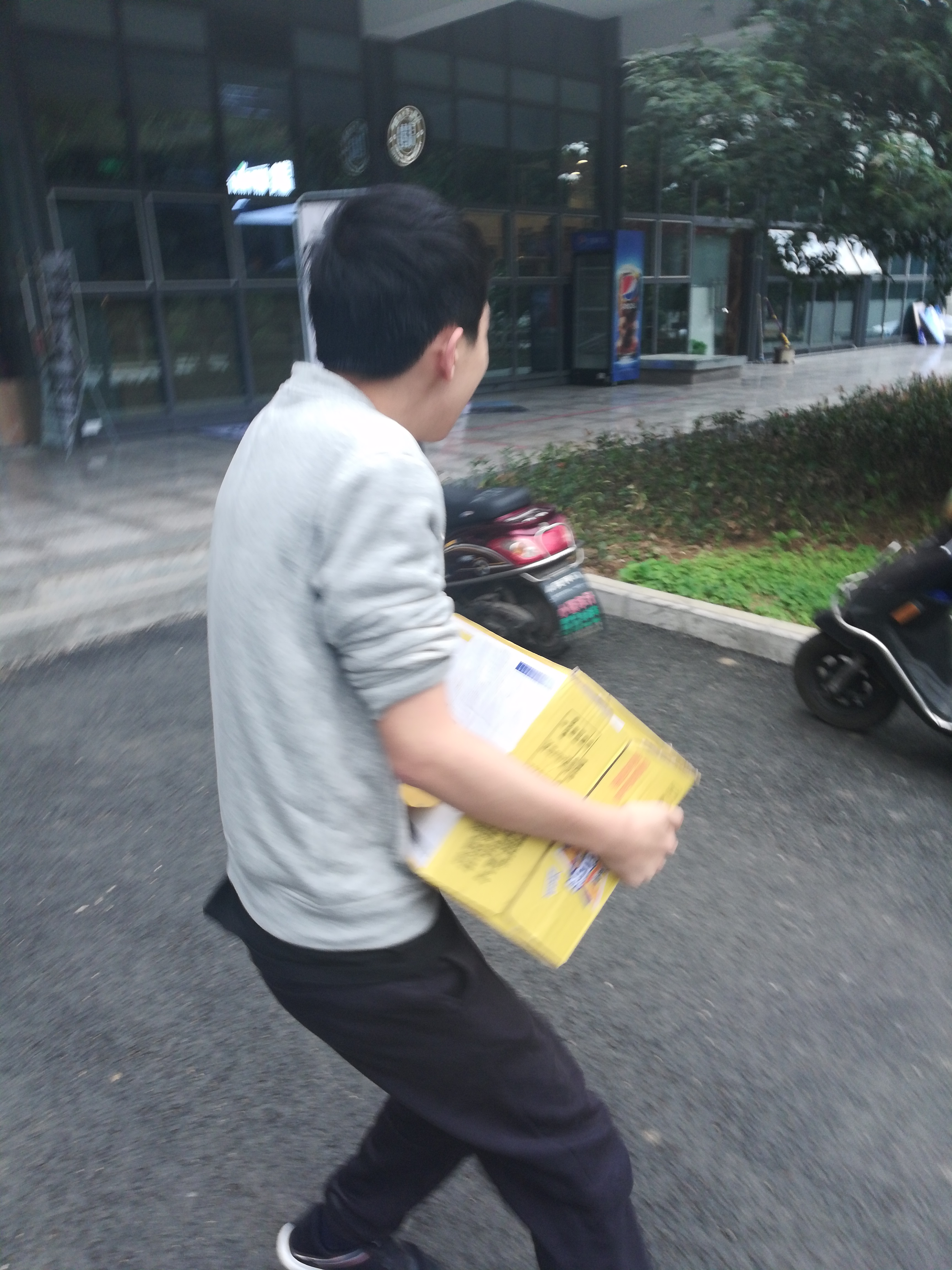动车比较晚，我们就分头打车去夫子庙周边吃晚饭。
和南宋御街好像呀，不过还要大很多呢。和集训队的同学一起逛古色古香的街区，是另一种浪漫呢（噗嗤。随便找了一家店，又点了鸭血粉丝和蟹黄包(#.#)。全程背着沉重的板子，手机也没电了，心累……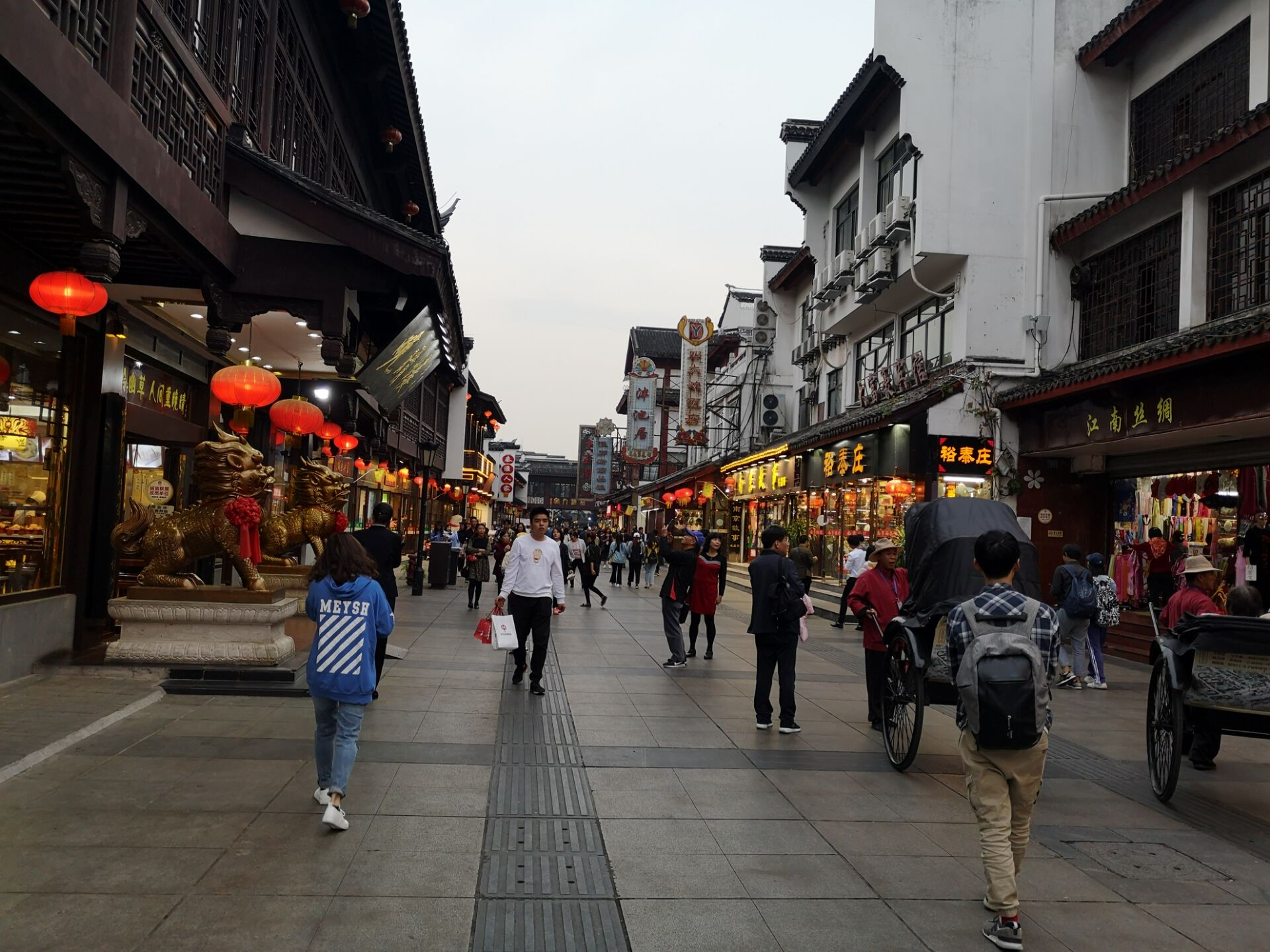等吃完出来后，天已经全黑了：亮起灯的古街实在是太美啦！灯光倒映在河里，脑海里立刻蹦出了《桨声灯影里的秦淮河》。哎呀，真想和小笨蛋一起坐着船吹吹风。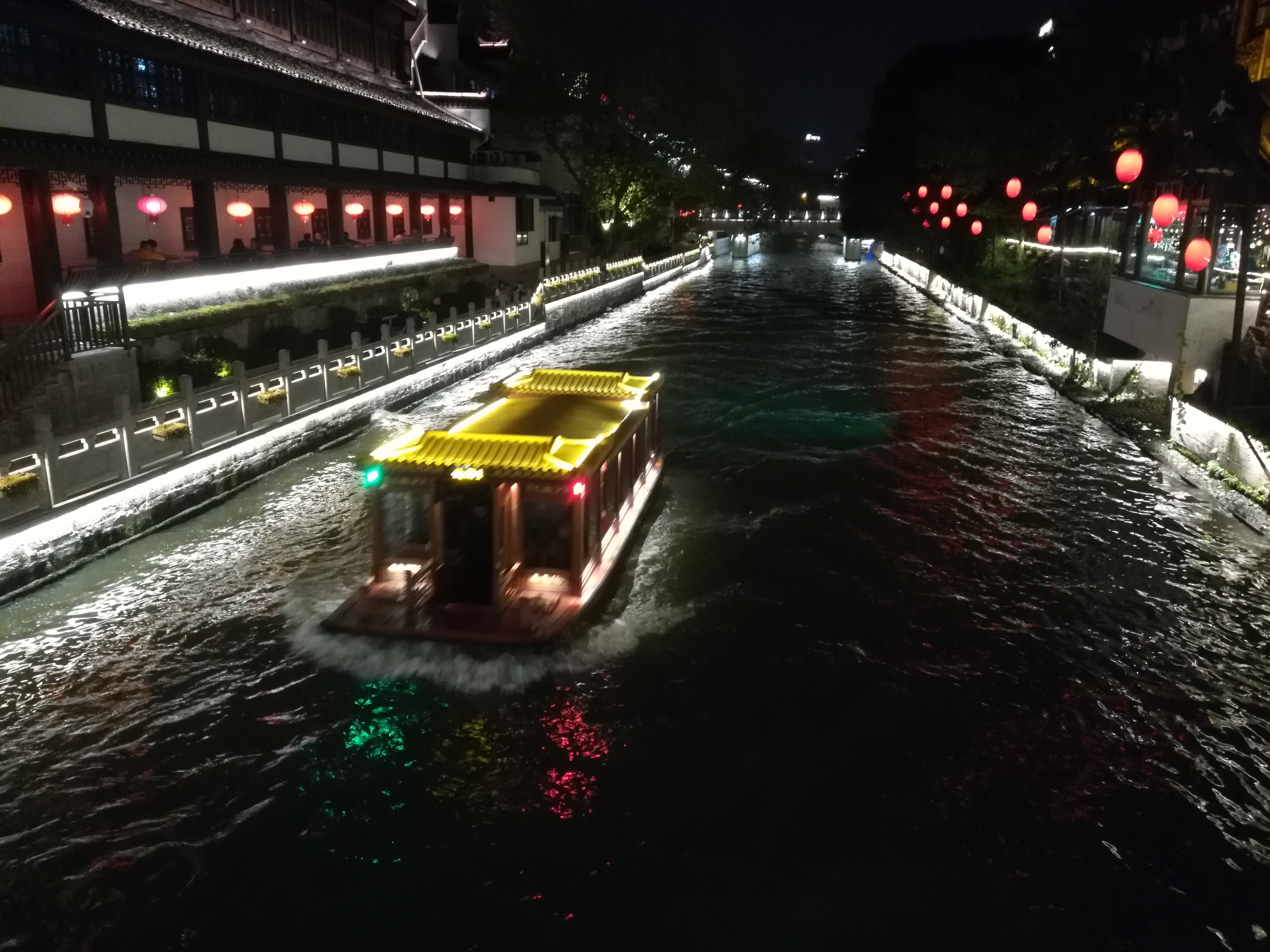$$cjb$$ 高考后来过这里，还很熟练地给我指了寄明信片的地方。突然替他难过，如此深情的男孩子却经受这种悲伤。想来想去不知道写什么好呢。在大家的催促下，匆匆涂了一些，还划来划去的￣□￣。能不能寄到呢？

回东站后，紫金港的小伙伴一起地铁回去。天下着小雨，微冷，到寝室已经十一点了。疯狂肝作业，启动！

## Summary

从一个寻求出线的队伍的角度评价：此次比赛按部就班，打得很稳重和踏实。前期和中后期遇到了一些小规模的失利，不过也没有太大的慌张，做到了及时调整。稳扎稳打，符合预期，赞一个。
从一个想在final上夺取名次（雾~）的队伍的角度评价：比赛较为糟糕，没有完全打出自己的实力。刚开场没有准确判断屠版的意义，竟然傻兮兮地无脑 $$A$$ 题的表。数列表也在手，$$G$$ 题打表打得迟了，直接强推式子的能力也不够。没有特别强的跟榜能力，套路不足，导致 $$B$$$$H$$ 都不会做。最后时刻决策较为紊乱，轮流上机手忙脚乱最终果然没过 $$E$$。总之很糟糕，很值得改进吧。

posted @ 2018-10-15 22:11  了491  阅读(977)  评论(3编辑  收藏  举报